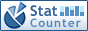Home PC Games Linux Windows Database Network Programming Server Mobile
 Home \ Programming \ Java, boolean operators & =, | = ^ = use - Use rfkill soft-switching and Bluetooth wireless capabilities in Linux (Linux) - Xmanager Remote Desktop login CentOS 6.5 (Linux) - Practical top command (Linux) - CentOS7 Kubernetes used on container management (Server) - System Safety: Windows and Linux platforms (Linux) - I like Linux Security (Linux) - To obtain installation package (RPM) under RHEL6 (Linux) - How to use nmap command in Linux (Linux) - Linux simple commands (Linux) - Revised OpenJDK Java Memory Model (Programming) - Linux netstat command to get started (Linux) - Linux Network Programming - raw socket instance: MAC Address Scanner (Programming) - Use Docker containers (Linux) - CentOS7 build GlusterFS (Linux) - numpy and SciPy installation under Python for scientific computing package (Linux) - Windows Desktop use VNC remote connect Linux (Linux) - High-performance JavaScript loops and flow control (Programming) - MongoDB simple replication configuration (Database) - Ubuntu 14.10 users to install Audio Recorder 1.5.7 (Linux) - Object-oriented language Java some of the basic features (Programming) Java, boolean operators & =, | = ^ = use Add Date : 2018-11-21 Today workmates code code review time to see a more fun writing. "Flag & = false;", at first glance, but also I felt he was wrong, but the program works, and quickly go to Baidu, look at the wording in the end is how one thing, it turned out that with the usual common - = + =, and so is the same, but usually use less of it, this really is a tool to enhance the Cock wire feelings, at least not to see this wording does not know what is the meaning of it. Code is as follows:   public class MainMethod {     public static void main (String [] args) {         boolean flag = true;         flag & = true;         System.out.println ( "true \ t & = \ ttrue \ t ==> \ t" + flag);         flag = true;         flag & = false;         System.out.println ( "true \ t & = \ tfalse \ t ==> \ t" + flag);         flag = false;         flag & = true;         System.out.println ( "false \ t & = \ ttrue \ t ==> \ t" + flag);         flag = false;         flag & = false;         System.out.println ( "false \ t & = \ tfalse \ t ==> \ t" + flag + "\ n");                    flag = true;         flag | = true;         System.out.println ( "true \ t | = \ ttrue \ t ==> \ t" + flag);         flag = true;         flag | = false;         System.out.println ( "true \ t | = \ tfalse \ t ==> \ t" + flag);         flag = false;         flag | = true;         System.out.println ( "false \ t | = \ ttrue \ t ==> \ t" + flag);         flag = false;         flag | = false;         System.out.println ( "false \ t | = \ tfalse \ t ==> \ t" + flag + "\ n");                    System.out.println ( "^ = same is true, except for the false");         flag = true;         flag ^ = true;         System.out.println ( "true \ t ^ = \ ttrue \ t ==> \ t" + flag);         flag = true;         flag ^ = false;         System.out.println ( "true \ t ^ = \ tfalse \ t ==> \ t" + flag);         flag = false;         flag ^ = true;         System.out.println ( "false \ t ^ = \ ttrue \ t ==> \ t" + flag);         flag = false;         flag ^ = false;         System.out.println ( "false \ t ^ = \ tfalse \ t ==> \ t" + flag);     } } Execution results are as follows: true & = true ==> true true & = false ==> false false & = true ==> false false & = false ==> false   true | = true ==> true true | = false ==> true false | = true ==> true false | = false ==> false   ^ = Same is true, except for the false true ^ = true ==> false true ^ = false ==> true false ^ = true ==> true false ^ = false ==> false More:
 CopyRight 2002-2022 newfreesoft.com, All Rights Reserved.